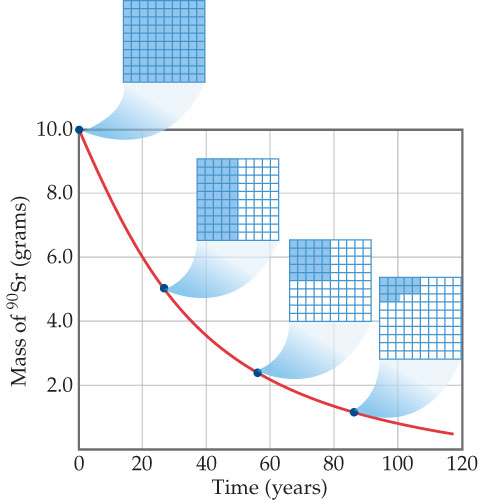# Problem: Decay of a 10.0-g sample of strontium-90 (t1/2 = 28.8 yr).The 10 ximes 10 grids show how much of the radioactive isotope remains aftervarious amounts of time.If we start with a 50.0-g sample, how much of it remains after three half-lives have passed?

###### FREE Expert Solution

In this problem, we are being asked to determine the mass of Strontium-90 remaining when after three half-lives have passed the 50.0 g of sample.

Recall that radioactive/nuclear decay of isotopes follows first-order kinetics, and the integrated rate law for first-order reactions is:

$\overline{){\mathbf{ln}}{\mathbf{\left[}\mathbf{N}\mathbf{\right]}}_{{\mathbf{t}}}{\mathbf{=}}{\mathbf{-}}{\mathbf{kt}}{\mathbf{+}}{\mathbf{ln}}{\mathbf{\left[}\mathbf{N}\mathbf{\right]}}_{{\mathbf{0}}}}$

where:

[N]t = concentration at time t

k = decay constant

t = time

[N]0 = initial concentration

Also, recall that half-life is the time needed for the amount of a reactant to decrease by 50% or one-half

The half-life of a first-order reaction is given by:

92% (138 ratings)###### Problem DetailsDecay of a 10.0-g sample of strontium-90 (t1/2 = 28.8 yr).The 10 x 10 grids show how much of the radioactive isotope remains aftervarious amounts of time.

If we start with a 50.0-g sample, how much of it remains after three half-lives have passed?